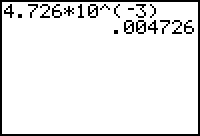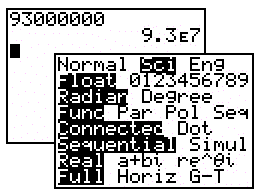Working with Scientific Notation

 In Normal Mode: (If a problem is already expressed IN scientific notation, it may be possible to simply enter the problem on the home screen in Normal mode to arrive at the answer.) Expressed in decimal notation, 4.726 x 10-3  is      (1) 0.004726         (2) 0.04726       (3) 472.6               (4) 4,726Note:  In Normal mode, if the answer cannot be displayed in 10 digits or the absolute value is less than .001, the answer will appear in scientific notation. In Scientific Mode: (If a problem asks for the answer to be in expressed in scientific notation, change the Mode to Sci (scientific mode). The distance from the Earth to the Sun is approximately 93 million miles.  A scientist would write that number as      (1) 9.3 x 106           (2) 9.3 x 107           (3) 93 x 107         (4) 93 x 1010In scientific mode (Sci), all answers will be expressed in scientific notation. Note:  The calculator is using the notation 9.3 E 7 to mean 9.3 x 107 . To insert the E into a calculation, press 2nd (comma) , The face of the calculator reads EE above the comma key, but only one E will appear on the screen for your scientific notation.  Can be used in either Normal or Sci mode. (Point of interest:  The double EE is used in Engineering Mode (Eng) where the powers of 10 are multiples of 3. The form is a = b x 10n; where b is a number from 1 to less than 1000, and n is a multiple of 3.)Finding Your Way Around TABLE of  CONTENTS